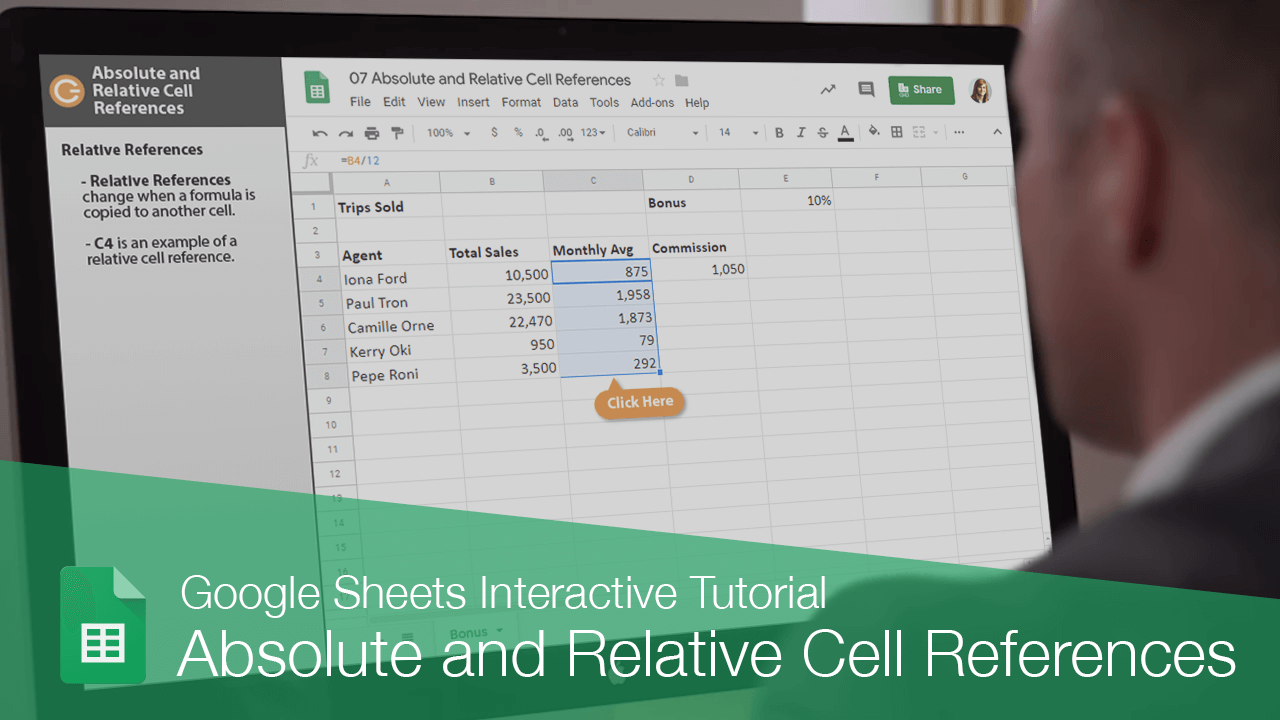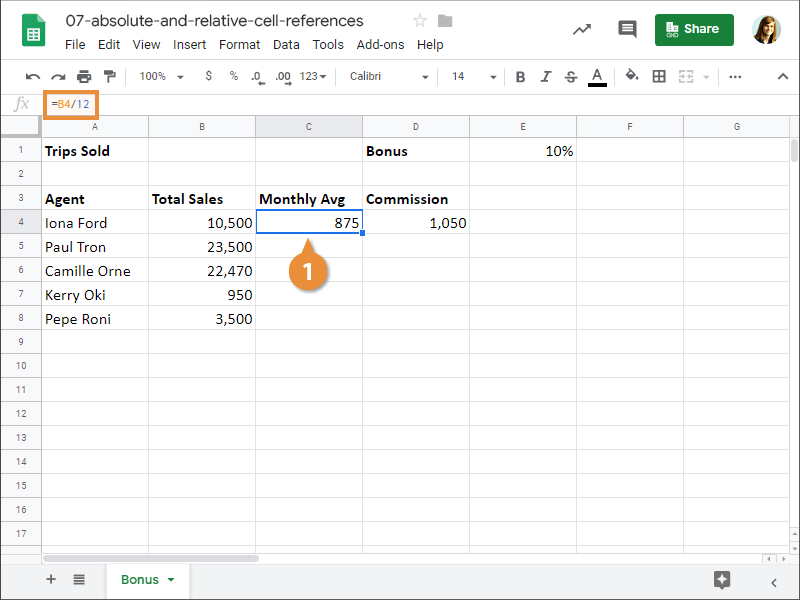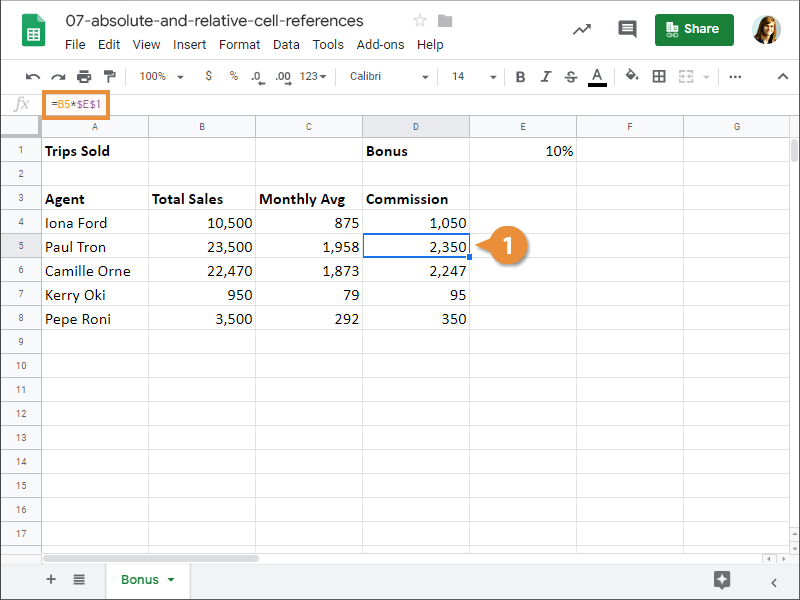Absolute and Relative Cell References | CustomGuide# Absolute and Relative Cell References

How to Use Absolute and Relative Cell References in Google SheetsFormulas can contain numbers, like 5 or 8, but more often they reference the contents of cells. A cell reference tells Sheets where to look for values you want to use in a formula. For example, the formula =A5+A6 adds the values in cells A5 and A6.

Using cell references is useful because if you change the values in the referenced cells, the formula result automatically updates using the new values. There are two different ways to refer to other cells while creating a formula—relative references and absolute references. Let's take a look at the difference between the two.

### Relative References

A relative cell reference is the default type.

• Relative References change when a formula is copied to another cell.
• B4 is an example of a relative cell reference.When a formula is copied, relative references update the formula based on the cell’s location.

### Absolute References

An absolute cell reference ensures that the formula is always refers to the same cell, even when it's copied and pasted.

• Absolute References remain constant no matter where they are copied.
• Add \$ symbols to make the reference absolute.
• \$E\$1 is an example of an absolute cell reference.• Absolute column and row reference (\$A\$1): The column and row remain constant no matter where the formula is pasted.
• Absolute column reference (\$A1): The column remains absolute no matter where the formula is pasted, but the row updates relatively.
• Absolute row reference (A\$1): The row remains absolute no matter where the formula is pasted, but the column updates relatively.